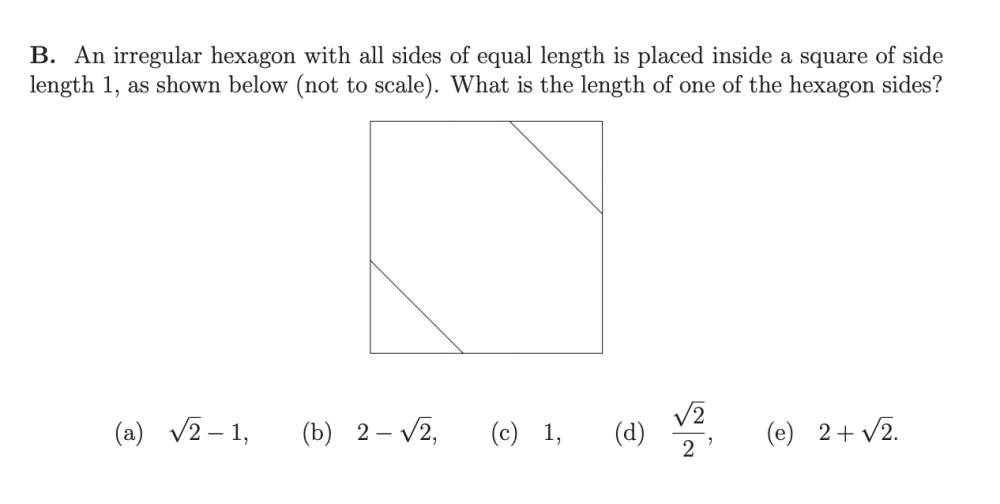# Finding length of a side of a hexagon inside a square.

## Recommended Posts

I found this question in an Oxford admissions quiz (2016 question 2) and was wondering how you would go about this. I am not applying for Oxford just thought that I might need to know how to do a question like this. Thanks!##### Share on other sites

You should at least attempt to show some effort in your OP so we know where you're getting stuck.  It is a simple problem that might appear in a middle-school algebra test, made all the simpler by the fact that it is multiple choice, so the answer is already sitting there in one of the 5 answers, most of which are already absurd.

##### Share on other sites

Set up an xy-coordinate system so that (0, 0) is  at the lower left.   Take the vertex in the middle of the bottom side to be (X, 0).  Then the vertex in the middle of the left side is (0, X).  The distance between those two points is sqrt{X^2+ X^2}= sqrt{2X^2}= Xsqrt{2} while the distance from the vertex in the middle of the bottom to the vertex on the right end if the bottom is 1- X.

Since those sides are the same length we have Xsqrt{2}= 1- X.    Xsqrt{2}+ X= (1+\sqrt{2})X= 1.  X= \frac{1}{1+ \sqrt{2}.

That's not one of the given answers  so "rationalize the denominator":  X= \frac{1}{1+ \sqrt{2}}\frac{1- \sqrt{2}}{1- \sqrt{2}}= \frac{1- \sqrt{2}}{1- 2}= \sqrt{2}- 1.

Edited by HallsofIvy

## Create an account

Register a new account